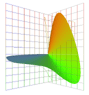Jim Worthey • Lighting & Color Research • jim@jimworthey.com • 301-977-3551 • 11 Rye Court, Gaithersburg, MD 20878-1901, USA

Question: Is Jozef Cohen’s Matrix R related to the Moore-Penrose pseudoinverse?

Projection Matrix R = A(A'A)−1A' , Derived from the
Pseudoinverse Formula, A+ = (A'A)−1A' .

Jozef Cohen derived the formula for the projection Matrix R without reference to the concept of pseudoinverse. However, projection Matrix R can be derived from the Moore-Penrose pseudoinverse, which is also familiar in color science1.

The Wikipedia article can be our reference on pseudoinverse: http://en.wikipedia.org/wiki/Moore-Penrose_pseudoinverse .

Matrix Transpose and Hermitian: The notation in the Wikipedia article clashes slightly with Cohen and Kappauf. The pseudoinverse formulas apply to matrices having complex numbers as elements. Then an asterisk, *, to the right of a matrix indicates the Hermitian operation. Given a matrix A, its Hermitian, A*, is found by taking the transpose of A, and then the complex conjugate of each element. For color vectors and related matrices, complex numbers are not used. Therefore, for our purposes,

A' (below or in Cohen's articles) = A* (in Wikipedia's pseudoinverse discussion)  .         (1)

Where a notation such as A* appears in the Wikipedia article, it can be read as meaning A-transpose, written as A'. The clash occurs because Cohen used the asterisk suffix, *, to indicate the fundamental metamer, which has nothing to do with transpose, or with complex numbers. In everything below, Cohen's notation is followed. The prime symbol, ', denotes matrix transpose, and the asterisk, *, denotes a vector projected into a subspace.

Recall the use and meaning of projection matrix R: Let L be any vector, such as the spectrum of a light. Let A be a matrix whose columns are linearly independent vectors, such as 3 color-matching functions. Then L* is defined to be the projection of L into the subspace of the columns of A. An equivalent statement is that L* is that linear combination of the columns of A which is a least-squares best fit to L. Matrix R is the one-step solution:

L* = R L   ,             (2)
where R is known to be:
R = A(A'A)−1A'      .             (3)

Restatement of the original question: A vector L is given, and we want to approximate it by a linear combination of the columns of A. The linear combination is specified by a coefficient vector u. Then

L  Au         .       (4)

The task is to solve for u. For concreteness, suppose that A has 31 rows and 3 columns, then Eq. (4) is a set of 31 equations in 3 unknowns, the unknowns being the components of u. The unknowns are overdetermined, so we seek a best fit solution for u. Cohen solved the least squares problem directly, but now we use the pseudoinverse.

Special case applies: The pseudoinverse of A is indicated as A+. In the special case that the columns of A are linearly independent, an explicit formula can be written:
A+ = (A' A)1 A' .     (5)

In color-related problems, the columns of A will be linearly independent, therefore Eq. (5) applies. Using A+ as an inverse in Eq. (4), we find
u = A+ L                .                  (6)
Then from Eq. (5), substitute the explicit formula for A+ :
u (A' A)1 A' L      .               (7)

The approximation to L in Eq. (4) is our L*, so substituting Eq. (7) into Eq. (4) gives

L* = Au = A (A' A)1 A' L        .         (8)

Comparing Eq. (8) to Eq. (2), we see

R = A(A'A)−1A'      ,             (9)

the same as Eq. (3), but now deduced by Eq. (5), (6), (7) and (8).

In short, Cohen's formula for R can be deduced from the formula for the pseudoinverse.

1. Brian A. Wandell and Joyce E. Farrell, “Water into Wine: Converting Scanner RGB to Tristimulus XYZ,” Proceedings of SPIE Vol.1909, pp. 92-100, (1993).